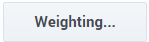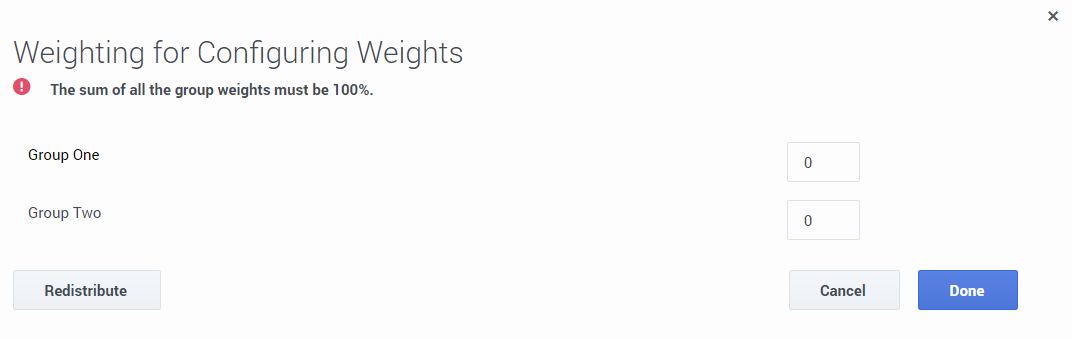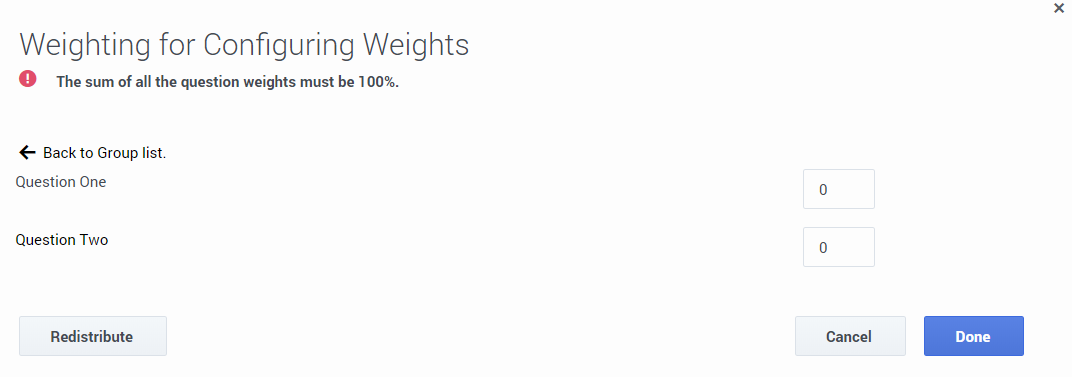# Configure Question Weights

Weights are assigned to Multiple Choice, Yes/No, Choose from list and Sliding Scale questions. The weights indicate the relative importance of each question and are used by the system to calculate the form's score.

Before you create a form consider the following.

• The sum of the group weights for each form must be 100%. If the sum of the group weights is not 100%, the form cannot be activated.
• The sum of the questions within each group must be 100%. If the sum of the questions weights within a group is not 100%, the form cannot be activated.
• When a question is marked as Required it must be answered.
• Each Multiple Choice and Choose from List answer should be assigned a weight.
• The weight for each answer, question, and/or group is either entered manually or distributed equally among the following by clicking the Redistribute button:
• Answers in a Multiple Choice question.
• Answers in a Choose from list question.
• All of the groups within a form.
• All of the questions within a group.

For a better understanding, refer to how weights are calculated for each of the following questions and answer types in the sections below.

## QM Questions and Answers

### Yes/No

The score for a Yes/No question is 100 if the answer is Yes and 0 if the answer is No.

### Multiple Choice

Each Multiple Choice answer can receive a weight from 0 to 100. The score associated with a Multiple Choice question is the weight of the selected answer.

For example - If a Multiple Choice question has four answers, the weights can be:

• Answer 1 = 0%
• Answer 2 = 40 %
• Answer 3 = 70 %
• Answer 4 = 100%

### Choose from list

Each Choose from list answer can receive a weight. The sum of all the weights must total 100%. The score associated with a Choose from list question is the sum of the weights of the selected answers.

For example - If a Choose from list question has four answers, the weights can be:

• Answer 1 = 20%
• Answer 2 = 40 %
• Answer 3 = 40 %
• Answer 4 = 0%

If the evaluator selects answers 1 and 3, the Choose from list question score is 60%.

### Sliding Scale

Each Scale question has a low (that is, minimum) value and high (that is, a maximum) value.

The score for the low value is 0 and the score for the high value is 100.

The Scale score is calculated as follows - (chosen value - low value) / (high value - low value) * 100%

For example:

The low value = 0 and the high value = 10.

If the evaluator selects 7, the score is (7 - 0) / (10 - 0) * 100 = 70%

### Free Form

Free form questions do not have a score and therefore they cannot be assigned a weight.

### Not Applicable (N/A), Not Required and Not Answered

A Yes/No question can include a N/A answer.

If a question is not marked as required the evaluator does not have to answer the question.

Selecting N/A or not answering a question will not affect the final form score.

### Auto Fail

When an answer marked as Auto Fail is selected, the form will automatically fail. The Yes/No, Multiple Choice, Choose from list questions can have answers with an Auto Fail answer, and the minimum Sliding Scale answer can be assigned the Auto Fail value.

If the evaluator selects an Auto Fail answer for one or more questions, the total score of the form will be 0. The scores of all remaining questions and groups will be calculated normally.

## Basic Scenarios

#### Scenario 1: A form has 2 groups. One group includes a N/A answer and the other group includes a question that was not answered.

Group One:

- 2 questions

- Group weight = 50%

 Question Answer Question Score (%) Question Weight Score Max Possible Score 1 80% 60% 24 30 2 N/A - 40% - -

Group One Score - 24 / 30 = 80%

Group Two:

- 3 questions

- Group weight = 50%

 Question Answer Question Score (%) Question Weight Score Max Possible Score 1 50% 60% 15 30 2 20% 20% 2 10 3 Not Answered - 20% -

Group Two Score - 17 / 40 = 42.5%

Total Form Score -  (80% * 50%) + (42.5% * 50%) = 61%

#### Scenario 2: A form with 2 groups where all questions are answered.

Group One:

- 2 questions

- Group weight = 50%

 Question Question Score (%) Question Weight Question's Score Absolute Question's Score Max Possible Score 1 100% 50% 100%*50%*50%=25% 25 25 2 80% 50% 80%*50%*50%=25% 20 25

Group One Score: 45 / 50 = 90%

Group Two:

- 3 questions

- Group weight = 50%

 Question Question Score (%) Question Weight Question's Score Absolute Question's Score Max Possible Score 1 50% 60% 50%*60%*50%=15% 15 30 2 20% 20% 20%*20%*50%=2% 2 10 3 100% 20% 100%*20%*50%=10% 10 10

Group One Score: 27 / 50 = 54%

Total Form Score -  (90% * 50%) + (54% * 50%) = 72%

#### Scenario 3: A form with 2 groups where all questions are either not answered or answered N/A. In this case, the group is removed from the total and will not affect the form score.

Group One:

- 2 questions

- Group weight = 50%

 Question Answer Question Score Question Weight Score 1 N/A - 50% - 2 N/A - 50% -

Group One Score: no score

Group Two:

- 3 questions

- Group weight = 50%

 Question Answer Question Score Question Weight Score Maximum Possible Score 1 50% 60% 15 30 2 20% 20% 2 10 3 Not Answered - 20%

Group One Score: 17 / 40 = 43%

Total Form Score -  43%

#### Scenario 4: A form where all the questions in the for are Free Form questions.

Free Form questions do not have a weight. For this reason, this form will not have a score. The message "no score" will appear and this form will not be included in the reports.

## Configure weights for a group, question or answer

1. Create a form with one or more groups of questions.
2. Click Weighting.... The Weighting for <form name> window appears.1. Enter a weight for each group in the fields provided or click Redistribute to distribute the weights equally among each group in the form.
2. Verify that the group weights total is 100.
3. Click the name of each group to see a list of the group's questions.1. Enter a weight for each question within the group, and verify that the total of the weights for the group's questions is 100 or click the Redistribute button to distribute the weights equally among each question within the group.
2. If you included a Multiple Choice or Choose from list question in your group, click the name of the question to see a list of the question's answer.
3. Enter a weight for each answer associated with the Multiple Choice or Choose from list question or click the Redistribute button to distribute the weights equally among each answer within the question.
4. Repeat steps 5-8 for each group in the form.
5. Save the form.